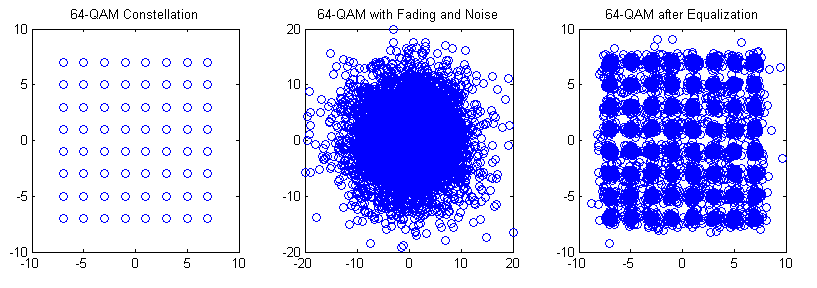# M-QAM Bit Error Rate in Rayleigh Fading

We have previously discussed the bit error rate (BER) performance of M-QAM in AWGN. We now discuss the BER performance of M-QAM in Rayleigh fading. The one-tap Rayleigh fading channel is generated from two orthogonal Gaussian random variables with variance of 0.5 each. The complex random channel coefficient so generated has an amplitude which is Rayleigh distributed and a phase which is uniformly distributed. As usual the fading channel introduces a multiplicative effect whereas the AWGN is additive.

The function “QAM_fading” has three inputs, ‘n_bits’, ‘M’, ‘EbNodB’ and one output ‘ber’. The inputs are the number of bits to be passed through the channel, the alphabet size and the Energy per Bit to Noise Power Spectral Density in dB respectively whereas the output is the bit error rate (BER).

```%%%%%%%%%%%%%%%%%%%%%%%%%%%%%%%%%%%%%%%%%%%%%%%%%%%%%%%%%%%%%%%%%%
% FUNCTION THAT CALCULATES THE BER OF M-QAM IN RAYLEIGH FADING
% n_bits: Input, number of bits
% M: Input, constellation size
% EbNodB: Input, energy per bit to noise power spectral density
% ber: Output, bit error rate
% Copyright RAYmaps (www.raymaps.com)
%%%%%%%%%%%%%%%%%%%%%%%%%%%%%%%%%%%%%%%%%%%%%%%%%%%%%%%%%%%%%%%%%%
function[ber]= QAM_fading(n_bits, M, EbNodB)
% Transmitter
k=log2(M);
EbNo=10^(EbNodB/10);
x=transpose(round(rand(1,n_bits)));
h1=modem.qammod(M);
h1.inputtype='bit';
h1.symbolorder='gray';
y=modulate(h1,x);

% Channel
Eb=mean((abs(y)).^2)/k;
sigma=sqrt(Eb/(2*EbNo));
w=sigma*(randn(n_bits/k,1)+1i*randn(n_bits/k,1));
h=(1/sqrt(2))*(randn(n_bits/k,1)+1i*randn(n_bits/k,1));
r=h.*y+w;

r=r./h;
h2=modem.qamdemod(M);
h2.outputtype='bit';
h2.symbolorder='gray';
h2.decisiontype='hard decision';
z=demodulate(h2,r);
ber=(n_bits-sum(x==z))/n_bits
return
%%%%%%%%%%%%%%%%%%%%%%%%%%%%%%%%%%%%%%%%%%%%%%%%%%%%%%%%%%%%%%%%%%
```The bit error rates of four modulation schemes 4-QAM, 16-QAM, 64-QAM and 256-QAM are shown in the figure above. All modulation schemes use Gray coding which gives a few dB of margin in the BER performance. As with the AWGN case each additional bit per symbol requires about 1.5-2 dB in signal to ratio to achieve the same BER.

Although not shown here similar behavior is observed for higher order modulation schemes such as 1024-QAM and 4096-QAM (the gap in the signal to noise ratio for the same BER is increased to about 5dB).

Lastly we explain some of the terms used above.